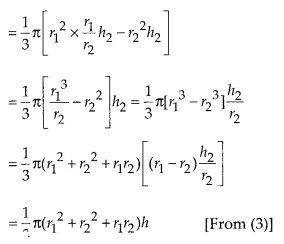In this article, we will share MP Board Class 10th Maths Book Solutions Chapter 13 Surface Areas and Volumes Ex 13.5 Pdf, These solutions are solved subject experts from the latest edition books.

## MP Board Class 10th Maths Solutions Chapter 13 Surface Areas and Volumes Ex 13.5

Question 1.
A copper wire, 3 mm in diameter, is wound about a cylinder whose length is 12 cm, and diameter 10 cm, so as to cover the curved surface of the cylinder. Find the length and mass of the wire, assuming the density of copper to be 8.88 g per cm3.
Solution:
Since, diameter of the cylinder = 10 cm 10
∴ Radius of the cylinder (r) = $$\frac{10}{2}$$ cm = 5cm
⇒ Length of wire in one round = 2πr
= 2 × 3.14 × 5 cm = 31.4 cm
∵ Diameter of wire = 3 mm = $$\frac{3}{10}$$ cm
∴ The thickness of cylinder covered in one round = $$\frac{3}{10}$$ cm
⇒ Number of rounds (turns) of the wire to cover 12 cm = $$\frac{12}{3 / 10}=12 \times \frac{10}{3}$$ = 40
∴ Length of wire required to cover the whole surface = Length of wire required to complete 40 rounds
= l = 40 × 31.4 cm = 1256 cm
Now, radius of the wire = $$\frac{3}{2}$$ mm = $$\frac{3}{20}$$ cm
∴ Volume of wire = πr2l
= 3.14 × $$\frac{3}{20} \times \frac{3}{20}$$ × 1256 cm3
∵ Density of wire = 8.88 g/cm3
∴ Mass of the wire = [Volume of the wire] × density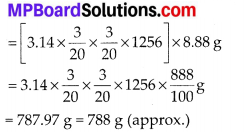Question 2.
A right triangle, whose sides are 3cm and 4cm (other than hypotenuse) is made to revolve about its hypotenuse. Find the volume and surface area of the double cone so formed. (Choose value of π as found appropriate).
Solution:
Let us consider the right ABAC, right angled at A such that AB = 3 cm, AC = 4 cm
∴ Hypotenuse BC = $$\sqrt{3^{2}+4^{2}}$$ = 5cm
Obviously, we have obtained two cones on the same base AA’ such that radius = DA or DA’.
Now,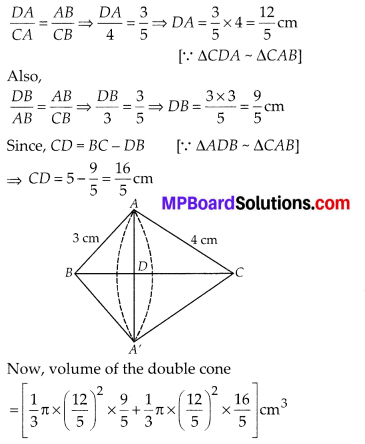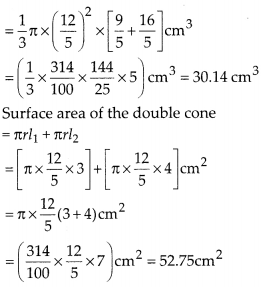Question 3.
A cistern, internally measuring 150 cm × 120 cm × 110 cm, has 129600 cm3 of water in it. Porous bricks are placed in the water until the cistern is full to the brim. Each brick absorbs one-seventeenth of its own volume of water. How many bricks can be put in without overflowing the water, each brick being 22.5 cm × 7.5 cm × 6.5 cm?
Solution:
Dimensions of the cistern are 150 cm, 120 cm and 100 cm.
∴ Volume of the cistern = 150 × 120 × 110 cm3 = 1980000 cm3
Volume of water contained in the cistern = 129600 cm3
∴ Free space (volume) which is not filled with water = (1980000 – 129600) cm3
= 1850400 cm3
Now, volume of one brick
= (22.5 × 7.5 × 6.5) cm3 = 1096.875 cm3
∴ Volume of water absorbed by one brick
= $$\frac{1}{17}$$ × 1096.875 cm3
Let n bricks can be put in the cistern.
∴ Volume of water absorbed by n bricks
= $$\frac{n}{17}$$ × 1096.875 cm3
∴ Volume occupied by n bricks = [free space in the cistern + volume of water absorbed by n bricks]
⇒ [n × 1096.875] = [1850400 + $$\frac{n}{17}$$(1096.875)]
⇒ 1096.875 n – $$\frac{n}{17}$$(1096.875) = 1850400
⇒ (n – $$\frac{n}{17}$$) × 1096.875 = 1850400
⇒ $$\frac{16}{17} n=\frac{1850400}{1096.875} \Rightarrow n=\frac{1850400}{1096.875} \times \frac{17}{16}$$
= 1792.4102 ≈ 1792
Thus, 1792 bricks can be put in the cistern.Question 4.
In one fortnight of a given month, there was a rainfall of 10 cm in a river valley. If the area of the valley is 97280 km2, show that the total rainfall was approximately equivalent to the addition to the norma water of three rivers each 1072 km long, 75 m wide and 3 m deep.
Solution:
Volume of three rivers = 3 {(Surface area of a river) × Depth}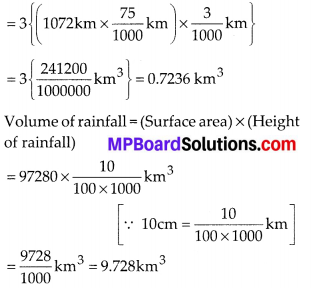Since 0.7236 km3 ≠ 9.728 km3
∴ The additional water in the three rivers is not equivalent to the rainfall.

Question 5.
An oil funnel made of tin sheet consists of a 10 cm long cylindrical portion attached to a frustum of a cone. If the total height is 22 cm, diameter of the cylindrical portion is 8 cm and the diameter of the top of the funnel is 18 cm, find the area of the tin sheet required to make the funnel (see figure).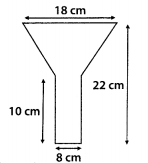Solution:
We have,
For the cylindrical part:
Diameter = 8 cm ⇒ Radius (r) = 4 cm
Height = 10 cm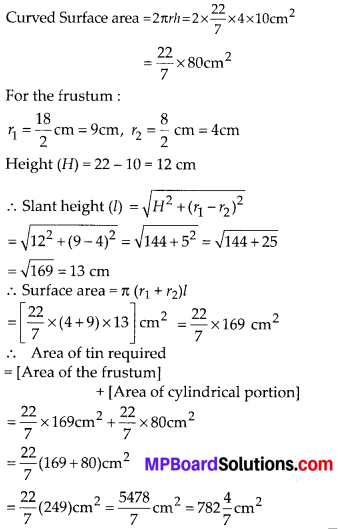Question 6.
Derive the formula for the curved surface area and total surface area of the frustum of a cone.
Solution: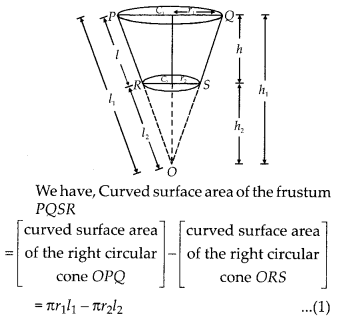Since, ∆OC1Q ~ ∆OC2S [By AA similarity]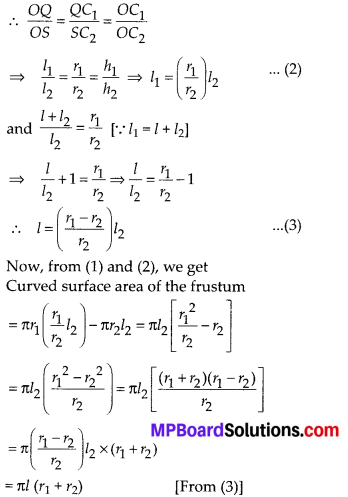Now, the total surface area of the frustum = (curved surface area) + (base surface area) + (top surface area)
= πl(r1 + r2) + πr22 + πr12 = π [(r1 + r2)l + r12 + r22]Question 7.
Derive the formula for the volume of the frustum of a cone.
Solution:
We have,
[Volume of the frustum RPQS] = [Volume of right circular cone OPQ] – [Volume of right circular cone ORS]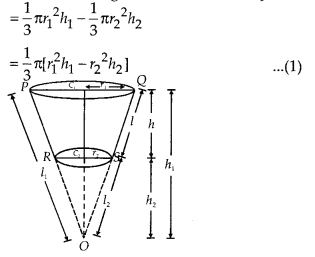Since, ∆OC1Q ~ ∆OC2S [By AA similarity]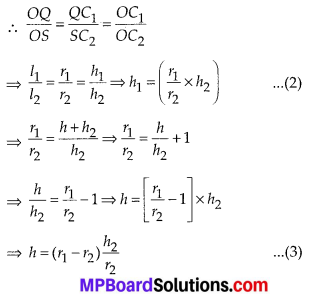From (1) and (2), we have
Volume of the frustum RPQS